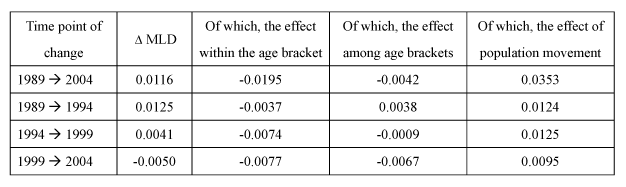Appended Note 3-8 Decomposition Analysis of Intertemporal Changes in Mean Logarithm Deviation

1. Method of decomposition analysis
The mean logarithm deviation (hereinafter referred to as MLD), an index used to capture the disparity of income distribution, has the characteristic that the disparity of the population can be decomposed into the disparity within each of certain groups which are obtained by dividing the population and the disparity among such groups. For instance, if the population consists of m groups in total and the average income of the gth bracket, its MLD and the ratio of it among the total population are expressed as Yg, MLDg, and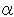g, respectively, then the MLD can be expressed as the following formula: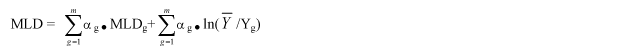If we use this property and conduct a decomposition analysis according to Mookherjee and Shorrocks (1982) with respect to the change in the MLD, then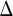MLD, the change in the MLD from time point 0 to time point 1, is expressed as follows: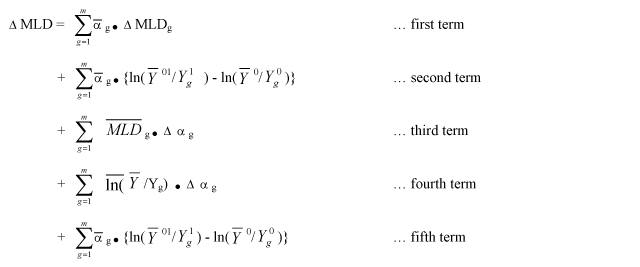Here, if the population is divided into m age brackets,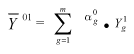stands for the average income of the gth age bracket at time point 1 after the age composition is fixed at time point 0, and the bar in the formula indicates the mean of each value at time point 0 and time point 1.
In this formula,
1) The first term on the right side represents the contribution by the change in the overall income disparity due to the change in the disparity within the same age bracket. (Effect within the age bracket)
2) The second term on the right side represents the contribution by the change in the overall income disparity due to the change in the disparity among different age brackets. (Effect among age brackets)
3) The remaining three terms represent the contribution by the change in the overall disparity due to the change in the population composition if the disparity at time point 0 is fixed. (Effect of population movement).

2. Results of the decomposition analysis
A decomposition analysis was conducted for the above 1. by using individual data of the National Survey of Family Income and Expenditure (total households) by the Ministry of Internal Affairs and Communications, and the following results were obtained: